# 10.8 Vectors  (Page 7/22)

 Page 7 / 22

## Verbal

What are the characteristics of the letters that are commonly used to represent vectors?

lowercase, bold letter, usually $\text{\hspace{0.17em}}u,v,w$

How is a vector more specific than a line segment?

What are $\text{\hspace{0.17em}}i\text{\hspace{0.17em}}$ and $\text{\hspace{0.17em}}j,$ and what do they represent?

They are unit vectors. They are used to represent the horizontal and vertical components of a vector. They each have a magnitude of 1.

What is component form?

When a unit vector is expressed as $⟨a,b⟩,$ which letter is the coefficient of the $\text{\hspace{0.17em}}i\text{\hspace{0.17em}}$ and which the $\text{\hspace{0.17em}}j?$

The first number always represents the coefficient of the $\text{\hspace{0.17em}}i,\text{\hspace{0.17em}}$ and the second represents the $\text{\hspace{0.17em}}j.$

## Algebraic

Given a vector with initial point $\text{\hspace{0.17em}}\left(5,2\right)\text{\hspace{0.17em}}$ and terminal point $\text{\hspace{0.17em}}\left(-1,-3\right),\text{\hspace{0.17em}}$ find an equivalent vector whose initial point is $\text{\hspace{0.17em}}\left(0,0\right).\text{\hspace{0.17em}}$ Write the vector in component form $⟨a,b⟩.$

Given a vector with initial point $\text{\hspace{0.17em}}\left(-4,2\right)\text{\hspace{0.17em}}$ and terminal point $\text{\hspace{0.17em}}\left(3,-3\right),\text{\hspace{0.17em}}$ find an equivalent vector whose initial point is $\text{\hspace{0.17em}}\left(0,0\right).\text{\hspace{0.17em}}$ Write the vector in component form $⟨a,b⟩.$

$〈7,-5〉$

Given a vector with initial point $\text{\hspace{0.17em}}\left(7,-1\right)\text{\hspace{0.17em}}$ and terminal point $\text{\hspace{0.17em}}\left(-1,-7\right),\text{\hspace{0.17em}}$ find an equivalent vector whose initial point is $\text{\hspace{0.17em}}\left(0,0\right).\text{\hspace{0.17em}}$ Write the vector in component form $⟨a,b⟩.$

For the following exercises, determine whether the two vectors $\text{\hspace{0.17em}}u\text{\hspace{0.17em}}$ and $\text{\hspace{0.17em}}v\text{\hspace{0.17em}}$ are equal, where $\text{\hspace{0.17em}}u\text{\hspace{0.17em}}$ has an initial point $\text{\hspace{0.17em}}{P}_{1}\text{\hspace{0.17em}}$ and a terminal point $\text{\hspace{0.17em}}{P}_{2}\text{\hspace{0.17em}}$ and $v$ has an initial point $\text{\hspace{0.17em}}{P}_{3}\text{\hspace{0.17em}}$ and a terminal point $\text{\hspace{0.17em}}{P}_{4}$ .

${P}_{1}=\left(5,1\right),{P}_{2}=\left(3,-2\right),{P}_{3}=\left(-1,3\right),\text{\hspace{0.17em}}$ and $\text{\hspace{0.17em}}{P}_{4}=\left(9,-4\right)$

not equal

${P}_{1}=\left(2,-3\right),{P}_{2}=\left(5,1\right),{P}_{3}=\left(6,-1\right),\text{\hspace{0.17em}}$ and $\text{\hspace{0.17em}}{P}_{4}=\left(9,3\right)$

${P}_{1}=\left(-1,-1\right),{P}_{2}=\left(-4,5\right),{P}_{3}=\left(-10,6\right),\text{\hspace{0.17em}}$ and $\text{\hspace{0.17em}}{P}_{4}=\left(-13,12\right)$

equal

${P}_{1}=\left(3,7\right),{P}_{2}=\left(2,1\right),{P}_{3}=\left(1,2\right),\text{\hspace{0.17em}}$ and $\text{\hspace{0.17em}}{P}_{4}=\left(-1,-4\right)$

${P}_{1}=\left(8,3\right),{P}_{2}=\left(6,5\right),{P}_{3}=\left(11,8\right),\text{\hspace{0.17em}}$ and ${P}_{4}=\left(9,10\right)$

equal

Given initial point $\text{\hspace{0.17em}}{P}_{1}=\left(-3,1\right)\text{\hspace{0.17em}}$ and terminal point $\text{\hspace{0.17em}}{P}_{2}=\left(5,2\right),\text{\hspace{0.17em}}$ write the vector $\text{\hspace{0.17em}}v\text{\hspace{0.17em}}$ in terms of $\text{\hspace{0.17em}}i\text{\hspace{0.17em}}$ and $\text{\hspace{0.17em}}j.\text{\hspace{0.17em}}$

Given initial point $\text{\hspace{0.17em}}{P}_{1}=\left(6,0\right)\text{\hspace{0.17em}}$ and terminal point $\text{\hspace{0.17em}}{P}_{2}=\left(-1,-3\right),\text{\hspace{0.17em}}$ write the vector $\text{\hspace{0.17em}}v\text{\hspace{0.17em}}$ in terms of $\text{\hspace{0.17em}}i\text{\hspace{0.17em}}$ and $\text{\hspace{0.17em}}j.\text{\hspace{0.17em}}$

$7i-3j$

For the following exercises, use the vectors u = i + 5 j , v = −2 i − 3 j ,  and w = 4 i j .

Find u + ( v w )

Find 4 v + 2 u

$-6i-2j$

For the following exercises, use the given vectors to compute u + v , u v , and 2 u − 3 v .

$u=⟨2,-3⟩,v=⟨1,5⟩$

$u=⟨-3,4⟩,v=⟨-2,1⟩$

$u+v=〈-5,5〉,u-v=〈-1,3〉,2u-3v=〈0,5〉$

Let v = −4 i + 3 j . Find a vector that is half the length and points in the same direction as $\text{\hspace{0.17em}}v.$

Let v = 5 i + 2 j . Find a vector that is twice the length and points in the opposite direction as $\text{\hspace{0.17em}}v.$

$-10i–4j$

For the following exercises, find a unit vector in the same direction as the given vector.

a = 3 i + 4 j

b = −2 i + 5 j

$-\frac{2\sqrt{29}}{29}i+\frac{5\sqrt{29}}{29}j$

c = 10 i j

$d=-\frac{1}{3}i+\frac{5}{2}j$

$-\frac{2\sqrt{229}}{229}i+\frac{15\sqrt{229}}{229}j$

u = 100 i + 200 j

u = −14 i + 2 j

$-\frac{7\sqrt{2}}{10}i+\frac{\sqrt{2}}{10}j$

For the following exercises, find the magnitude and direction of the vector, $\text{\hspace{0.17em}}0\le \theta <2\pi .$

$⟨0,4⟩$

$⟨6,5⟩$

$|v|=7.810,\theta =39.806°$

$⟨2,-5⟩$

$⟨-4,-6⟩$

$|v|=7.211,\theta =236.310°$

Given u = 3 i − 4 j and v = −2 i + 3 j , calculate $\text{\hspace{0.17em}}u\cdot v.$

Given u = − i j and v = i + 5 j , calculate $\text{\hspace{0.17em}}u\cdot v.$

$-6$

Given $\text{\hspace{0.17em}}u=⟨-2,4⟩\text{\hspace{0.17em}}$ and $\text{\hspace{0.17em}}v=⟨-3,1⟩,\text{\hspace{0.17em}}$ calculate $\text{\hspace{0.17em}}u\cdot v.$

Given u $=⟨-1,6⟩$ and v $=⟨6,-1⟩,$ calculate $\text{\hspace{0.17em}}u\cdot v.$

$-12$

## Graphical

For the following exercises, given $\text{\hspace{0.17em}}v,\text{\hspace{0.17em}}$ draw $v,$ 3 v and $\text{\hspace{0.17em}}\frac{1}{2}v.$

$⟨2,-1⟩$

$⟨-1,4⟩$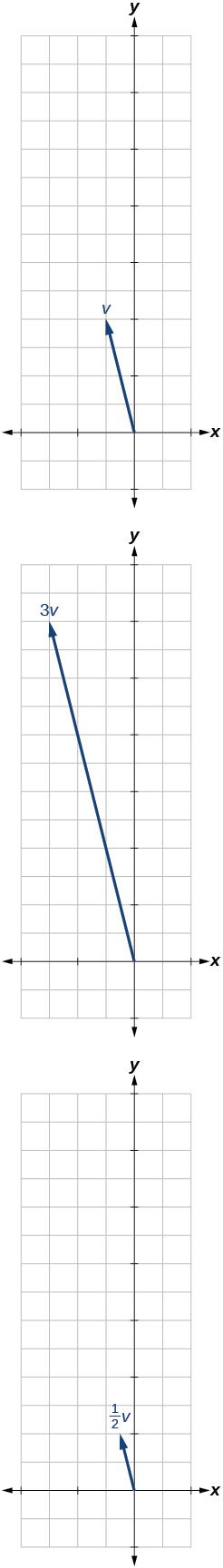$⟨-3,-2⟩$

For the following exercises, use the vectors shown to sketch u + v , u v , and 2 u .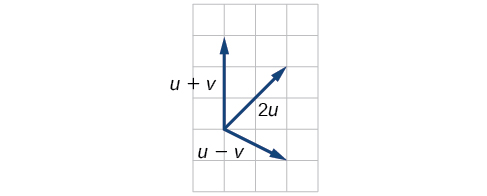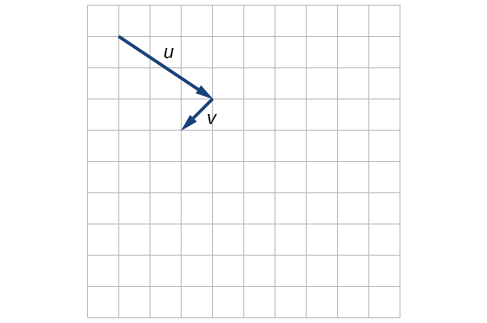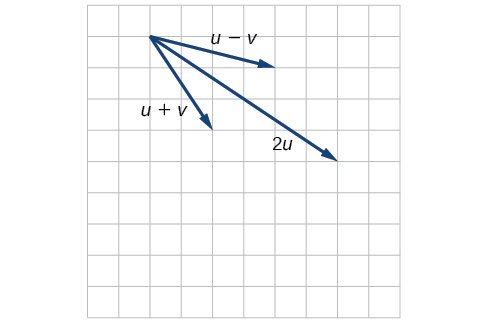For the following exercises, use the vectors shown to sketch 2 u + v .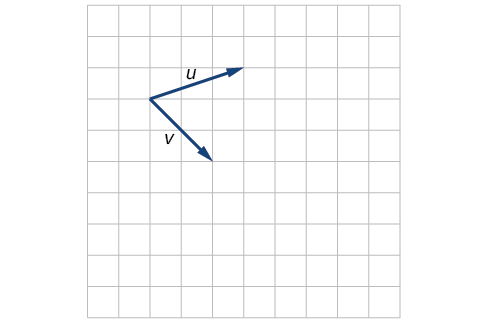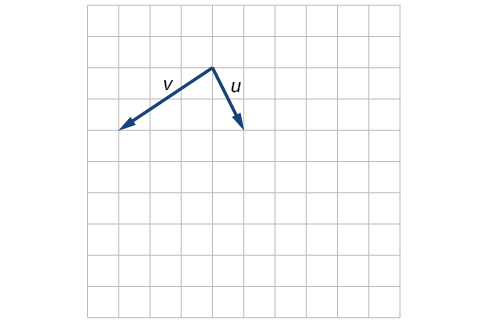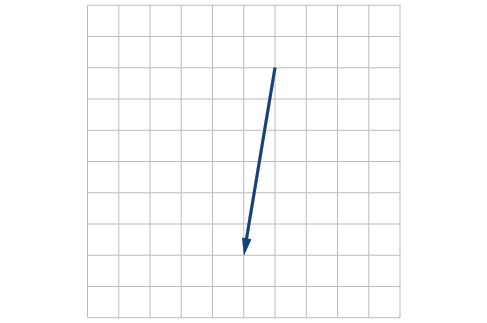For the following exercises, use the vectors shown to sketch u − 3 v .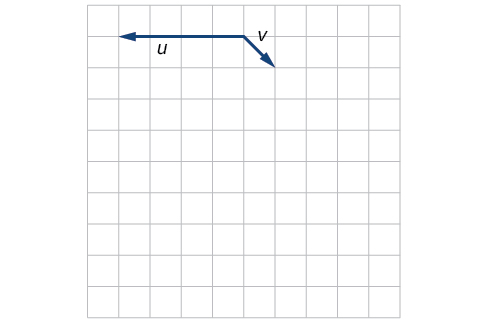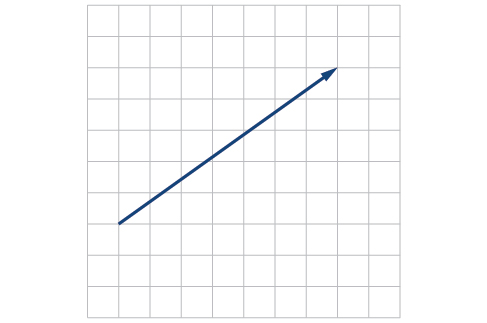For the following exercises, write the vector shown in component form.

A laser rangefinder is locked on a comet approaching Earth. The distance g(x), in kilometers, of the comet after x days, for x in the interval 0 to 30 days, is given by g(x)=250,000csc(π30x). Graph g(x) on the interval [0, 35]. Evaluate g(5)  and interpret the information. What is the minimum distance between the comet and Earth? When does this occur? To which constant in the equation does this correspond? Find and discuss the meaning of any vertical asymptotes.
The sequence is {1,-1,1-1.....} has
how can we solve this problem
Sin(A+B) = sinBcosA+cosBsinA
Prove it
Eseka
Eseka
hi
Joel
June needs 45 gallons of punch. 2 different coolers. Bigger cooler is 5 times as large as smaller cooler. How many gallons in each cooler?
7.5 and 37.5
Nando
find the sum of 28th term of the AP 3+10+17+---------
I think you should say "28 terms" instead of "28th term"
Vedant
the 28th term is 175
Nando
192
Kenneth
if sequence sn is a such that sn>0 for all n and lim sn=0than prove that lim (s1 s2............ sn) ke hole power n =n
write down the polynomial function with root 1/3,2,-3 with solution
if A and B are subspaces of V prove that (A+B)/B=A/(A-B)
write down the value of each of the following in surd form a)cos(-65°) b)sin(-180°)c)tan(225°)d)tan(135°)
Prove that (sinA/1-cosA - 1-cosA/sinA) (cosA/1-sinA - 1-sinA/cosA) = 4
what is the answer to dividing negative index
In a triangle ABC prove that. (b+c)cosA+(c+a)cosB+(a+b)cisC=a+b+c.
give me the waec 2019 questionsByByByByByBy